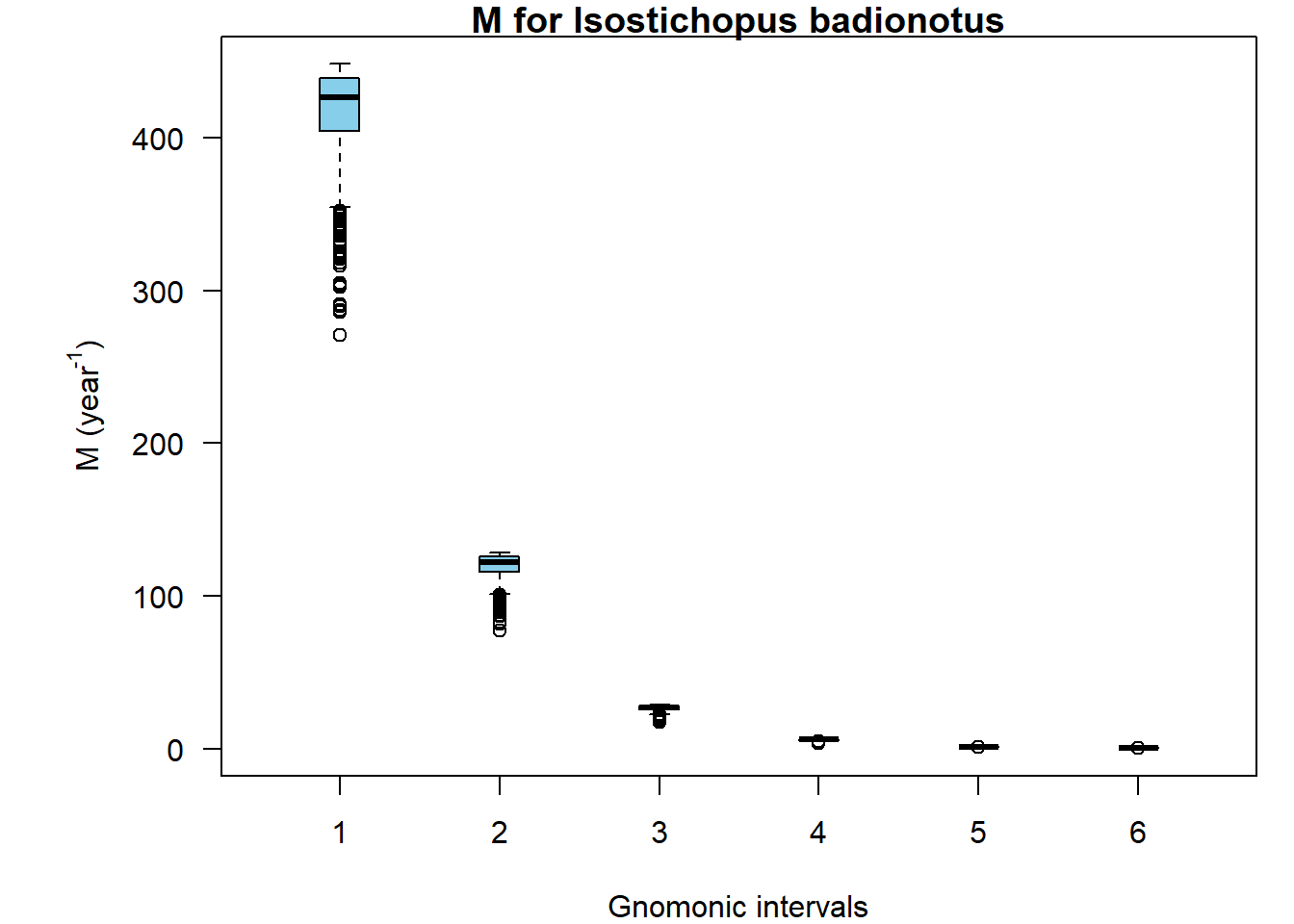This vignette introduces you to gnomonicM package and provides a way to estimate Natural Mortality (M) throughout the life history of species, mainly fish and invertebrates.

## Install package

You can install from CRAN:

install.packages("gnomonicM")

Or the development version from github:

# install.packages("devtools")
devtools::install_github("ejosymart/gnomonicM")

After that, call the package:

library("gnomonicM")

## 1. Natural mortality (M) via deterministic method

For estimating M we will use the data provided by Caddy (1996) based on two species with: (i) seven gnomonic intervals, (ii) egg stage duration of 2 days, (iii) a longevity of one year (365 days), (iv) a mean lifetime fecundity (MLF) of 200000 (high fecundity, hf) and 135 eggs (low fecundity, lf), and (v) initial constant proportionality ($$\alpha$$) value of 2.

model_hf <- gnomonic(nInterval   = 7,
eggDuration = 2,
longevity   = 365,
fecundity   = 200000,
a_init      = 2)
## --------------------------------------------------------
##
## No additional information. You are only considering the egg stage duration = 2
##
## --------------------------------------------------------
model_lf <- gnomonic(nInterval   = 7,
eggDuration = 2,
longevity   = 365,
fecundity   = 135,
a_init      = 2)
## --------------------------------------------------------
##
## No additional information. You are only considering the egg stage duration = 2
##
## --------------------------------------------------------

If you have additional information related to the duration of the other gnomonic intervals, you could provide them via the argument addInfo. For example, if we assume that the duration of the second and fifth gnomonic interval is equal to 4 and 40 days respectively, we will have to include this information as follows:

modelAddInfo <- gnomonic(nInterval   = 7,
eggDuration = 2,
addInfo     = c(4, NA, NA, 40, NA, NA),
longevity   = 365,
fecundity   = 200000,
a_init      = 2)

The NA values in the argument addInfo must be written to complete the length of the vector. In this case the length of the addInfo vector is equal to nInterval - 1.

## 2. Natural mortality (M) via stochastic method: the Pacific chub mackerel (Scomber japonicus) case

The function to be used to estimate M is gnomonicStochastic. The previous estimation of natural mortality, which was based on the gnomonic function, did not include any measures of deviation. In this method we calculated these measures assuming that the main source of uncertainty and variability was the mean lifetime fecundity (MLF).

We have included three different distribution function via the argument distr. It could be normal: distr = 'normal', uniform: distr = 'uniform', and triangular: distr = 'triangle'. Once you have chosen a particular distribution function, you must to include information related with the minimum, maximum, mean and standard deviation (sd) of MLF.

We will use the information reported by the chub mackerel, based on an (i) eight gnomonic intervals, (ii) egg stage duration of 2.33 days (56 hours), (iii) a longevity of eight years (2920 days), (iv) a mean lifetime fecundity (MLF) of 78174 [11805 - 144543] and 28978 [7603 - 53921] eggs assuming uniform distribution, and (v) initial constant proportionality ($$\alpha$$) value of 2. We simulated 1000 estimates of natural mortality for each gnomonic intervals and estimated mean mortality rate ($$\bar{M_i}$$), the confidence interval, and the standard deviation ($$\sigma_{i}$$).

model_cm_hf <- gnomonicStochastic(nInterval     = 8,
eggDuration   = 2.33,
longevity     = 2920,
distr         = "uniform",
min_fecundity = 11805,
max_fecundity = 144543,
niter         = 1000,
a_init        = 2)
## --------------------------------------------------------
##
## No additional information. You are only considering the egg stage duration = 2.33
##
## --------------------------------------------------------
##
##  "You are using a 'uniform distribution' for fecundity."
model_cm_lf <- gnomonicStochastic(nInterval     = 8,
eggDuration   = 2.33,
longevity     = 2920,
distr         = "uniform",
min_fecundity = 7603,
max_fecundity = 53921,
niter         = 1000,
a_init        = 2)
## --------------------------------------------------------
##
## No additional information. You are only considering the egg stage duration = 2.33
##
## --------------------------------------------------------
##
##  "You are using a 'uniform distribution' for fecundity."

## 3. Different distributions in MLF

In this section, we test different distribution functions in the MLF via the argument distr. There are three options: distr = 'normal', distr = 'uniform' and distr = 'triangle'. You must include particular information related to the minimum, maximum, mean, and standard deviation (sd) of MLF based on the particular distribution function.

modelUniformAddInfo <- gnomonicStochastic(nInterval     = 7,
eggDuration   = 2,
addInfo       = c(4, NA, NA, 40, NA, NA),
longevity     = 365,
distr         = "uniform",
min_fecundity = 100000,
max_fecundity = 300000,
niter         = 1000,
a_init        = 2)
##  "You are using a 'uniform distribution' for fecundity."
modelNormal <- gnomonicStochastic(nInterval     = 7,
eggDuration   = 2,
longevity     = 365,
distr         = "normal",
fecundity     = 200000,
sd_fecundity  = 50000,
niter         = 1000,
a_init        = 2)
## --------------------------------------------------------
##
## No additional information. You are only considering the egg stage duration = 2
##
## --------------------------------------------------------
##
##  "You are using a 'normal distribution' for fecundity."
modelTriangle <- gnomonicStochastic(nInterval     = 7,
eggDuration   = 2,
longevity     = 365,
distr         = "triangle",
fecundity     = 200000,
min_fecundity = 100000,
max_fecundity = 300000,
niter         = 1000,
a_init        = 2)
## --------------------------------------------------------
##
## No additional information. You are only considering the egg stage duration = 2
##
## --------------------------------------------------------
##
##  "You are using a 'triangular distribution' for fecundity."
plot(modelUniformAddInfo, main = "Uniform distribution in MLF, with additional information")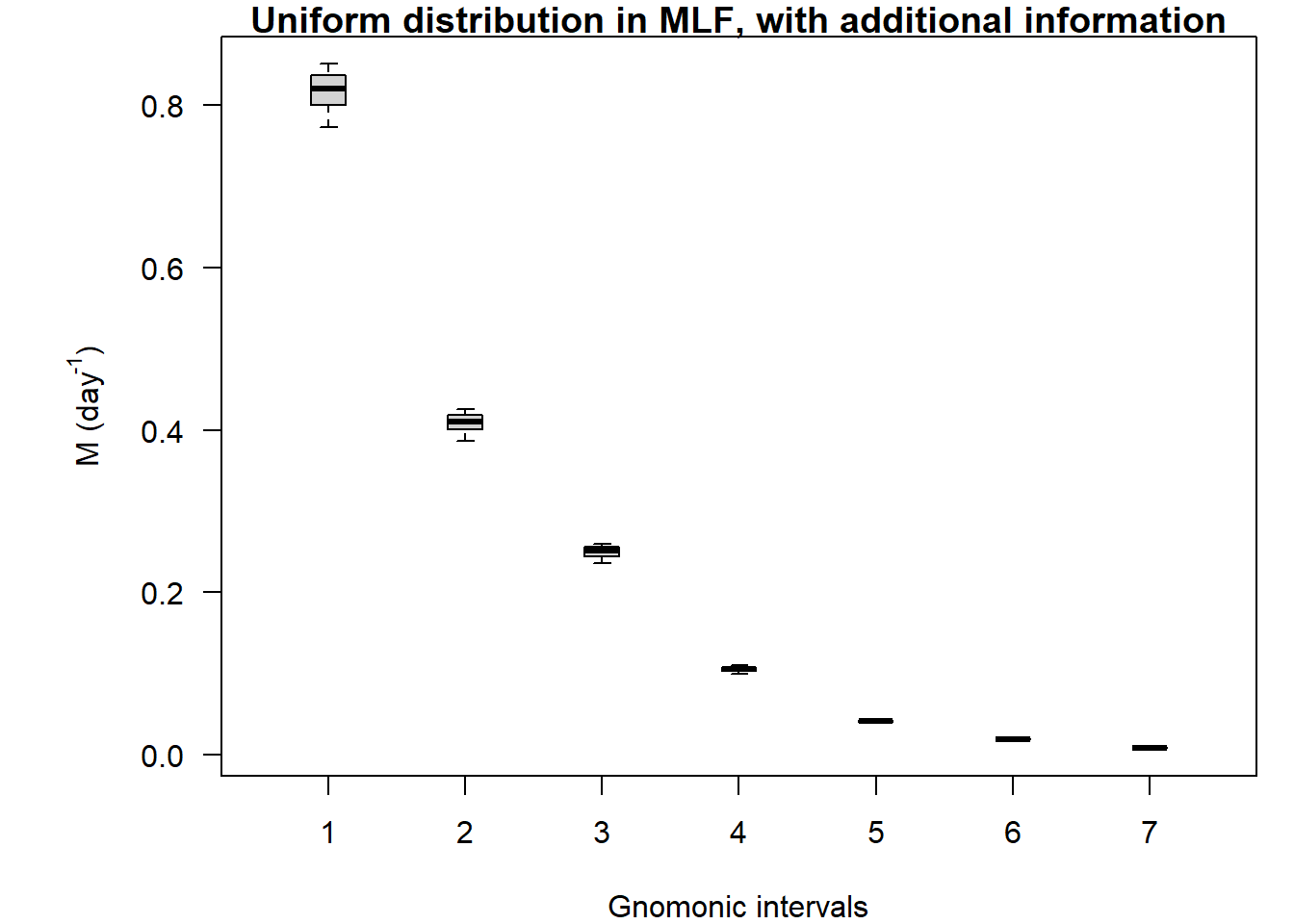plot(modelNormal, main = "Normal distribution in MLF")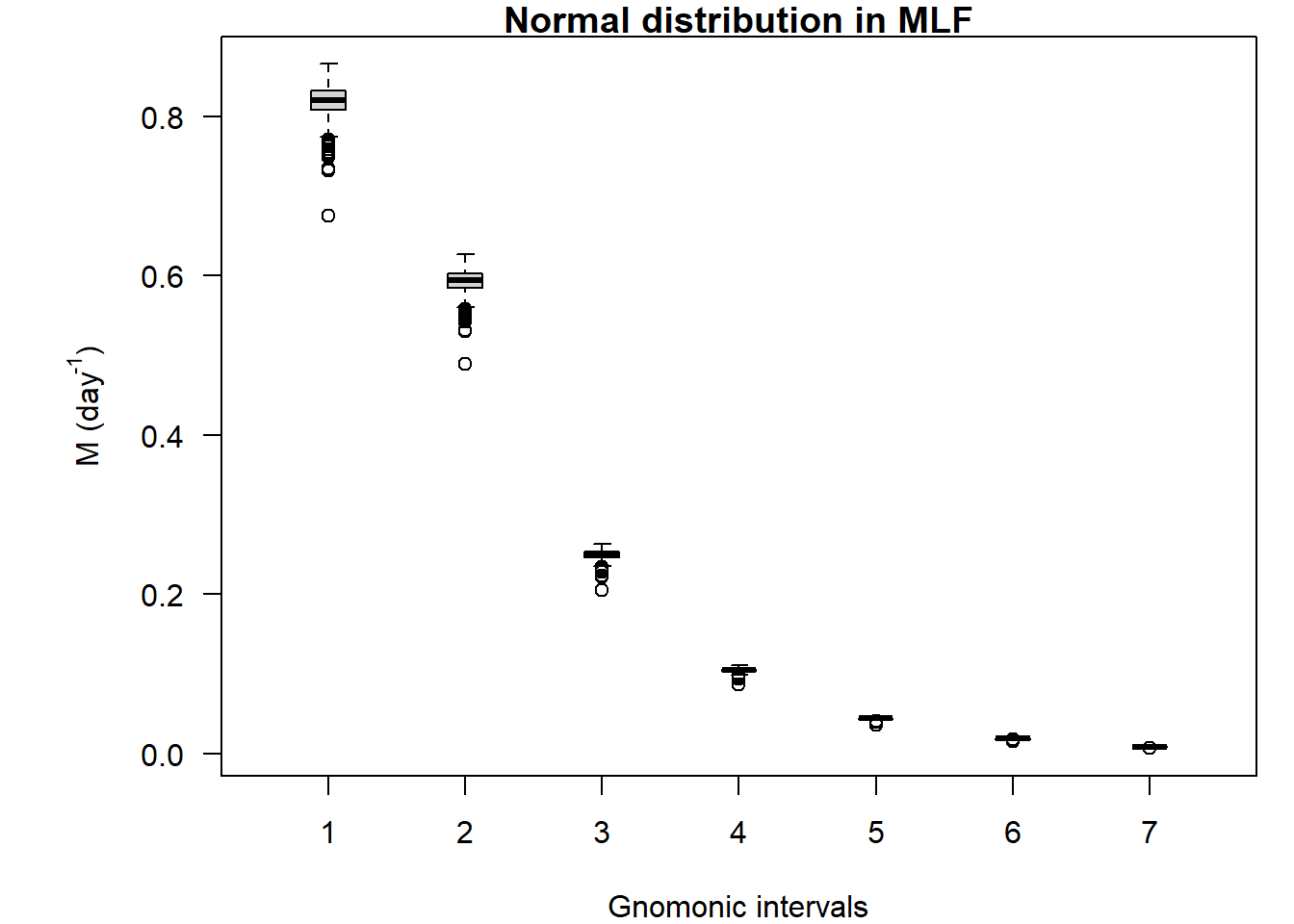plot(modelTriangle, main = "Triangular distribution in MLF")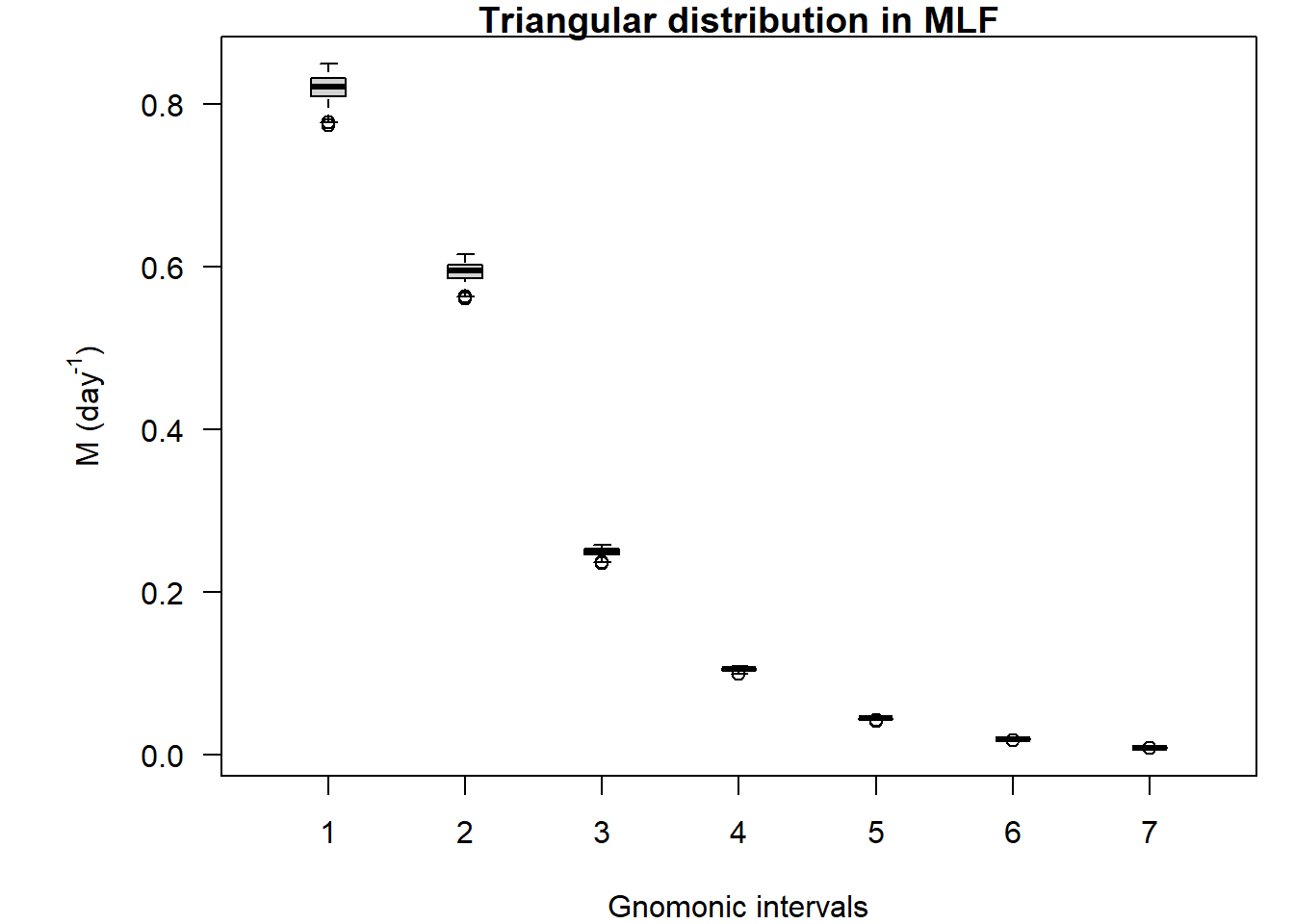## 4. Testing in other species

This section shows the applications to published data that used the gnomonic model (see, Ramírez-Rodríguez & Arreguín-Sánchez (2003); Martínez-Aguilar et al (2005); Giménez-Hurtado et al. (2009); Martínez-Aguilar et al. (2010); Aranceta-Garza et al. (2016); Romero-Gallardo et al. (2018)). It gave the chance to assess the approach in different taxa (fish, invertebrates) and life-history (demersal, pelagic, benthic, short, and large life span).

Farfantopenaeus <-  gnomonic(nInterval   = 7,
eggDuration = 1.5,
longevity   = 480,
fecundity   = 500000,
a_init      = 1)
## --------------------------------------------------------
##
## No additional information. You are only considering the egg stage duration = 1.5
##
## --------------------------------------------------------
Vannamei <- gnomonic(nInterval   = 7,
eggDuration = 0.54,
longevity   = 365,
fecundity   = 265000,
a_init      = 3)
## --------------------------------------------------------
##
## No additional information. You are only considering the egg stage duration = 0.54
##
## --------------------------------------------------------
Sardinops <- gnomonicStochastic(nInterval     = 10,
eggDuration   = 2.5,
longevity     = 2555,
min_fecundity = 646763,
max_fecundity = 1090678,
niter         = 1000,
a_init        = 2)
## --------------------------------------------------------
##
## No additional information. You are only considering the egg stage duration = 2.5
##
## --------------------------------------------------------
##
##  "You are using a 'uniform distribution' for fecundity."
Epinephelus <- gnomonicStochastic(nInterval     = 11,
eggDuration   = 2,
longevity     = 7300,
min_fecundity = 102000,
max_fecundity = 573500,
niter         = 1000,
a_init        = 2)
## --------------------------------------------------------
##
## No additional information. You are only considering the egg stage duration = 2
##
## --------------------------------------------------------
##
##  "You are using a 'uniform distribution' for fecundity."
Dosidicus <- gnomonicStochastic(nInterval     = 5,
eggDuration   = 6,
longevity     = 438,
min_fecundity = 813000,
max_fecundity = 25887000,
niter         = 1000,
a_init        = 2)
## --------------------------------------------------------
##
## No additional information. You are only considering the egg stage duration = 6
##
## --------------------------------------------------------
##
##  "You are using a 'uniform distribution' for fecundity."
Isostichopus <- gnomonicStochastic(nInterval     = 6,
eggDuration   = 2,
longevity     = 3650,
min_fecundity = 13500,
max_fecundity = 5062490,
niter         = 1000,
a_init        = 2)
## --------------------------------------------------------
##
## No additional information. You are only considering the egg stage duration = 2
##
## --------------------------------------------------------
##
##  "You are using a 'uniform distribution' for fecundity."
par(mar=c(5.1, 4.1, 6, 2.1))
plot(Farfantopenaeus, main = "M for Farfantopenaeus duorarum", dayUnits = FALSE)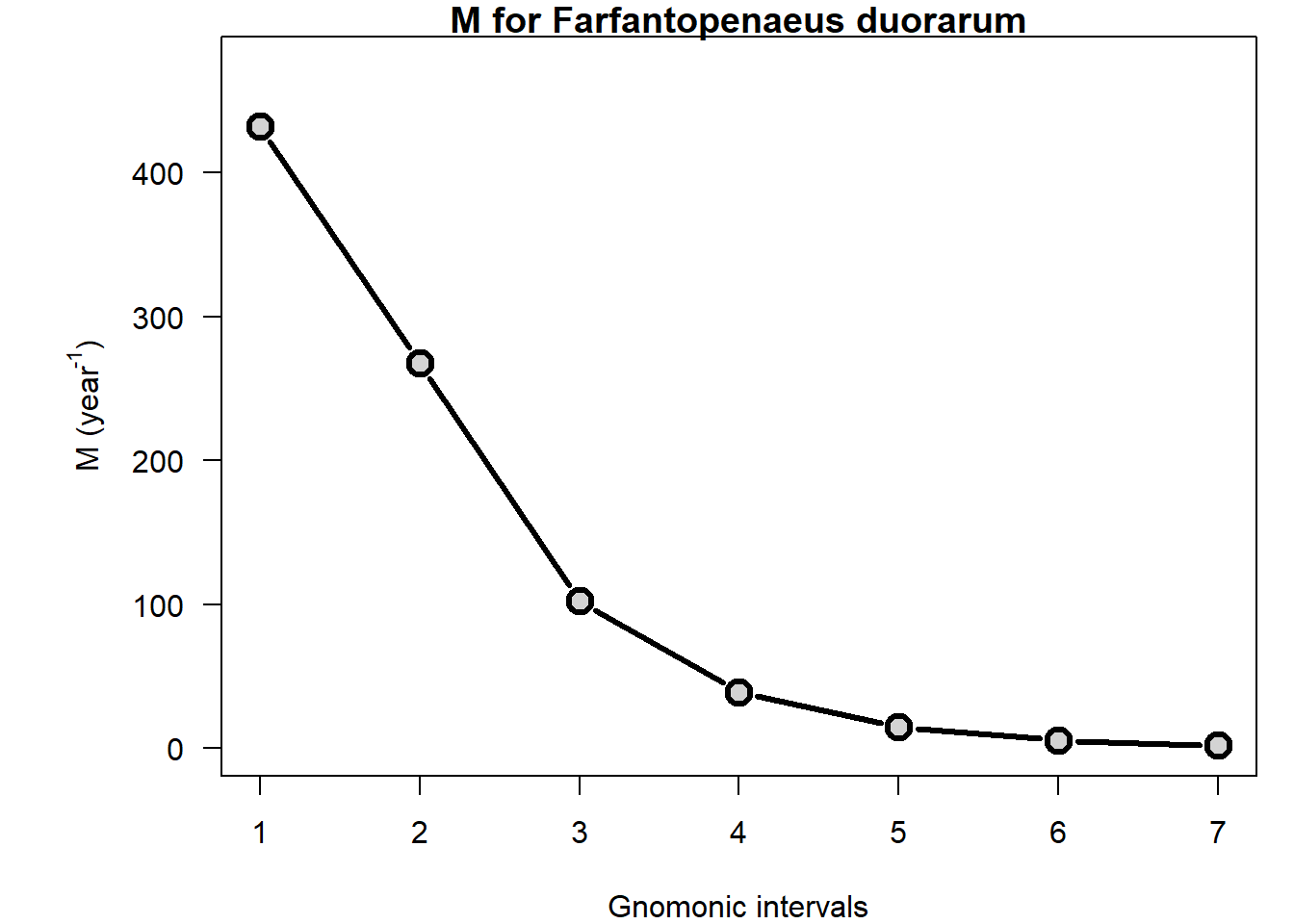plot(Vannamei, main = "M for Penaeus vannamei", col = "darkred", dayUnits = FALSE)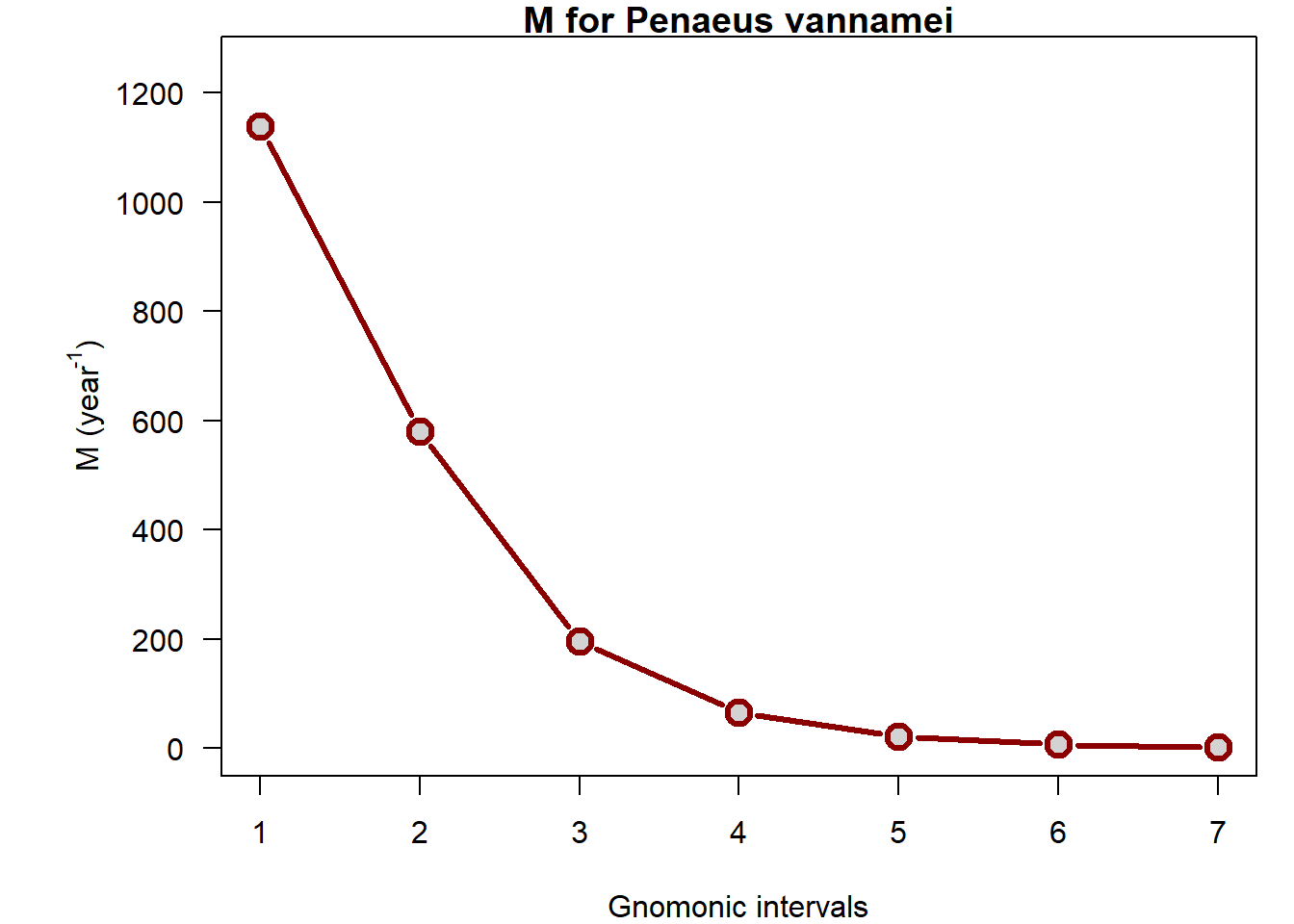plot(Sardinops, main = "M for Sardinops caeruleus", col = "blue")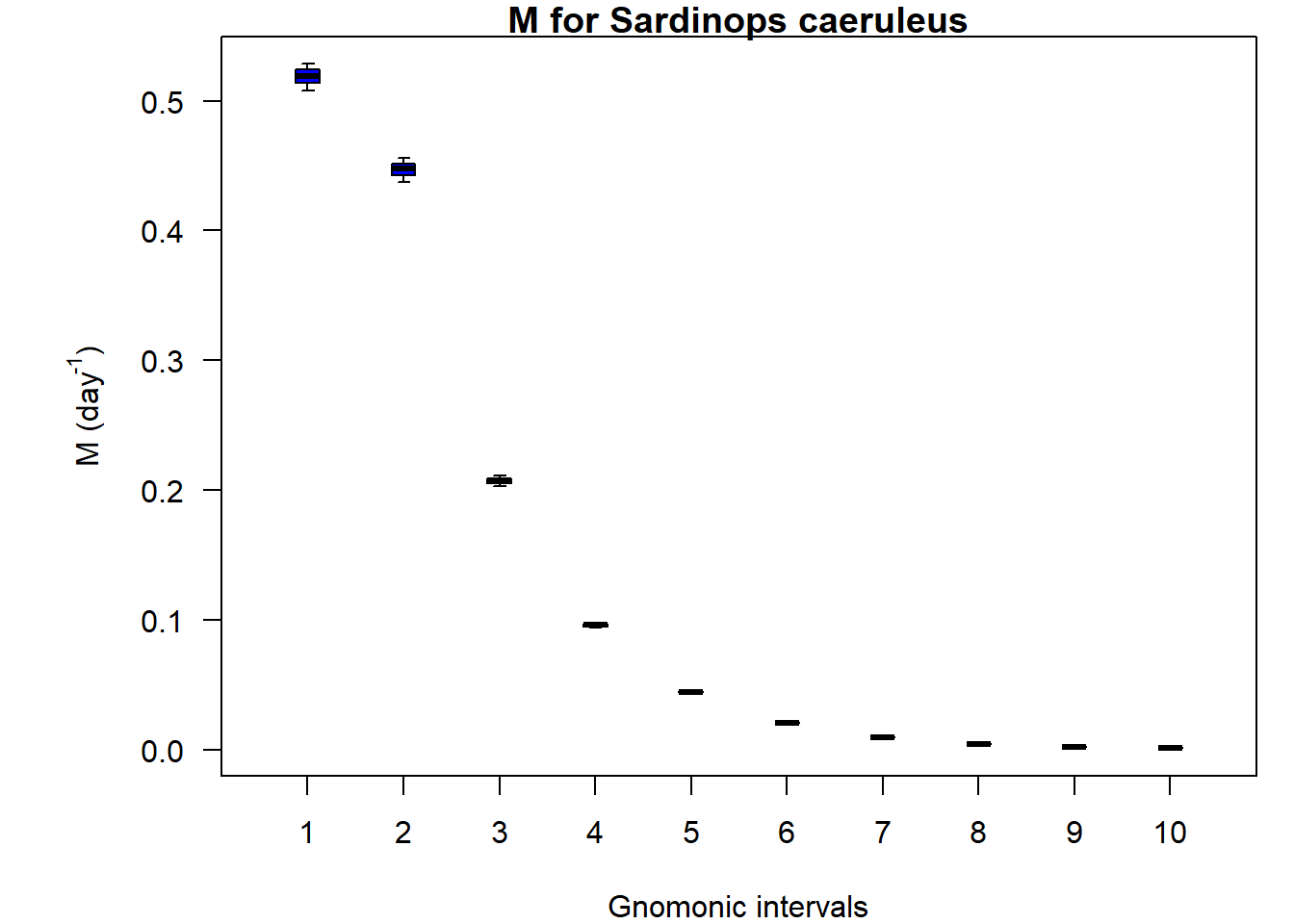plot(Epinephelus, main = "M for Epinephelus morio", col = "darkgreen", dayUnits = FALSE)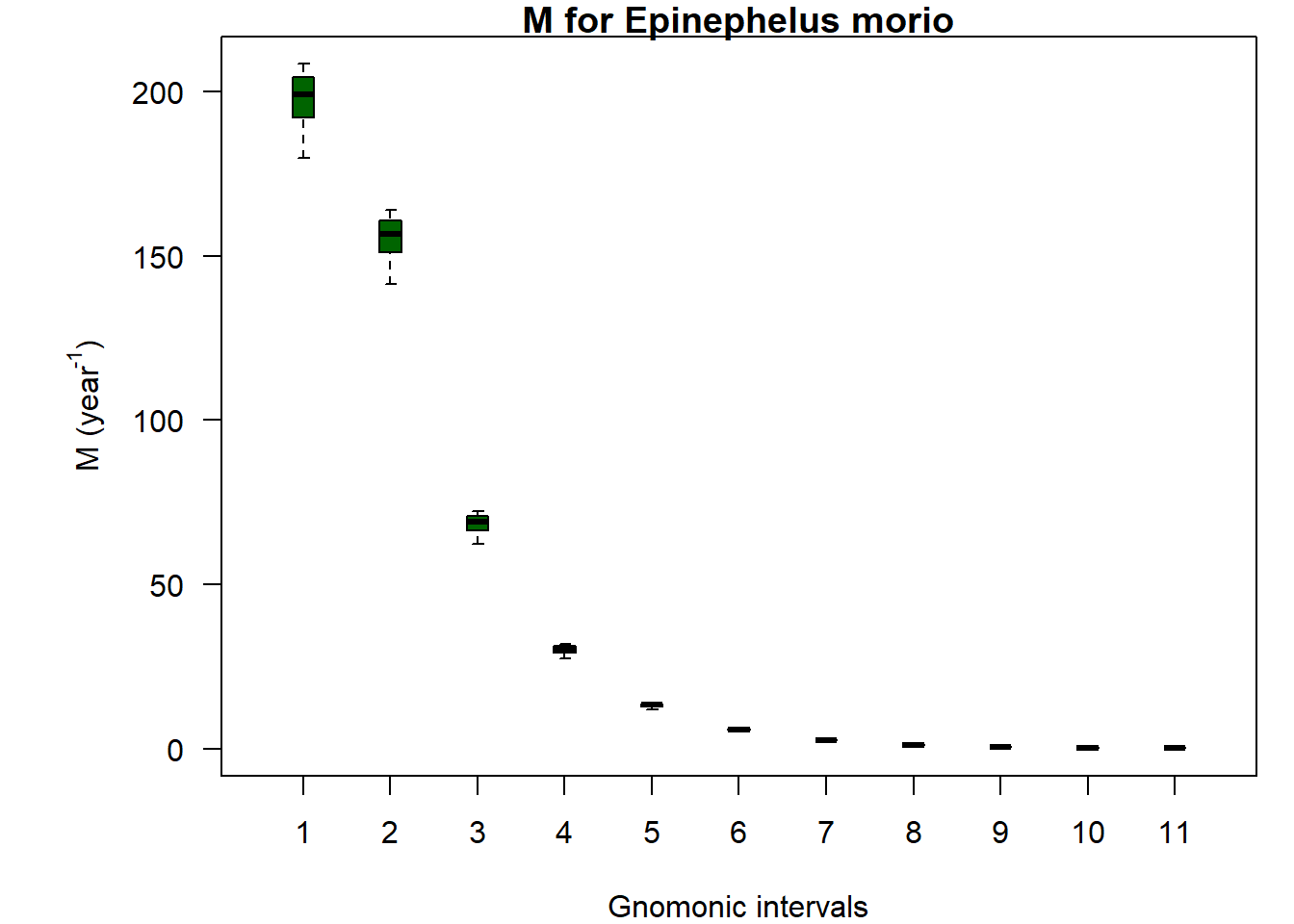plot(Dosidicus, main = "M for Dodisicus gigas", col = "purple", dayUnits = FALSE)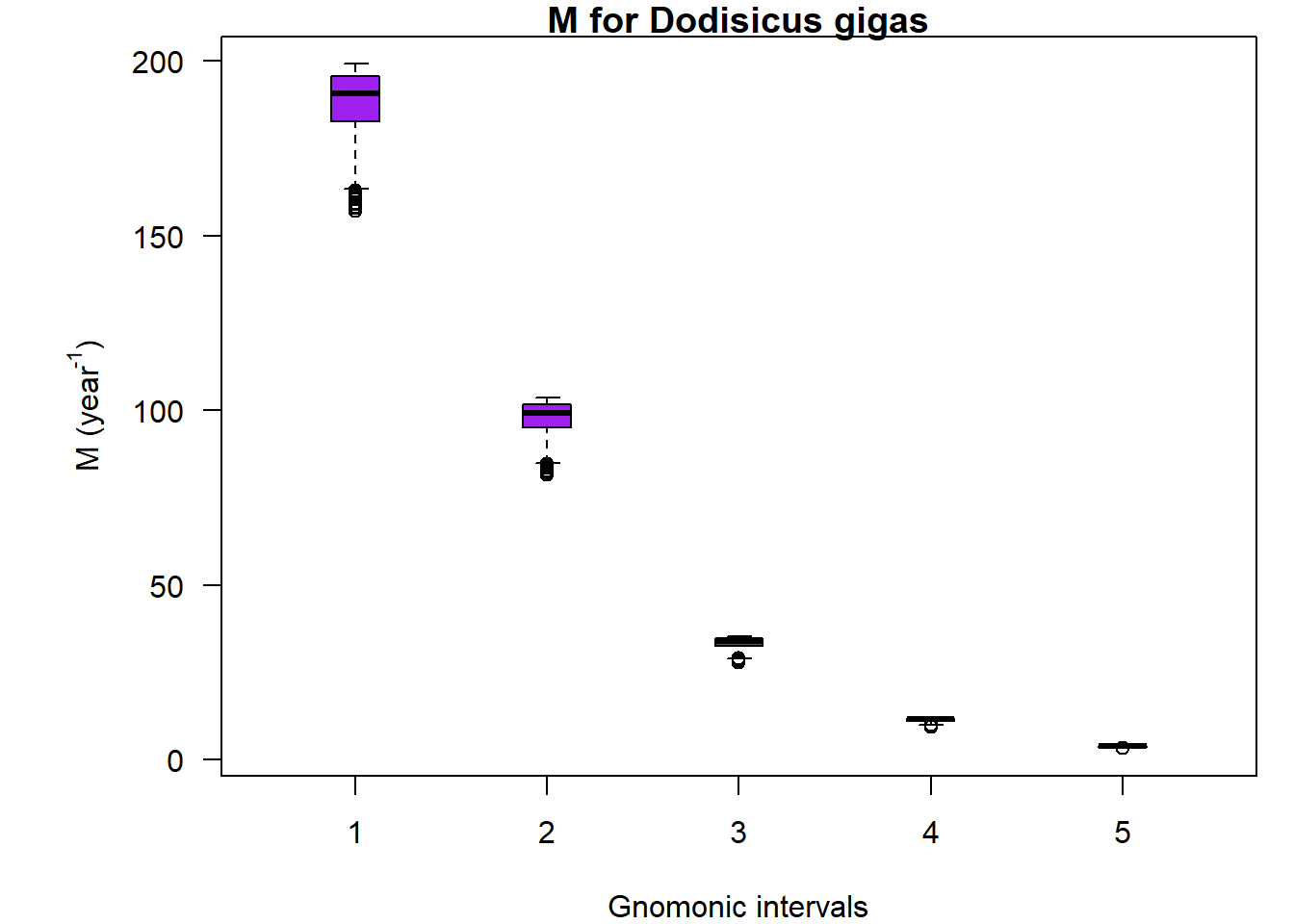plot(Isostichopus, main = "M for Isostichopus badionotus", col = "skyblue", dayUnits = FALSE)